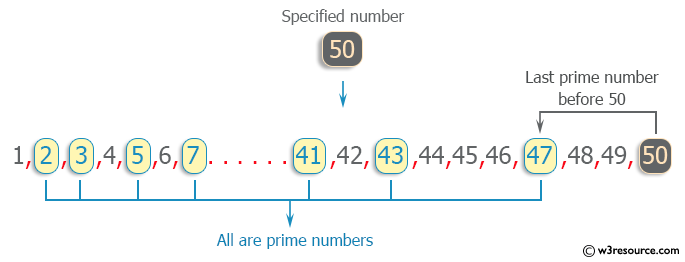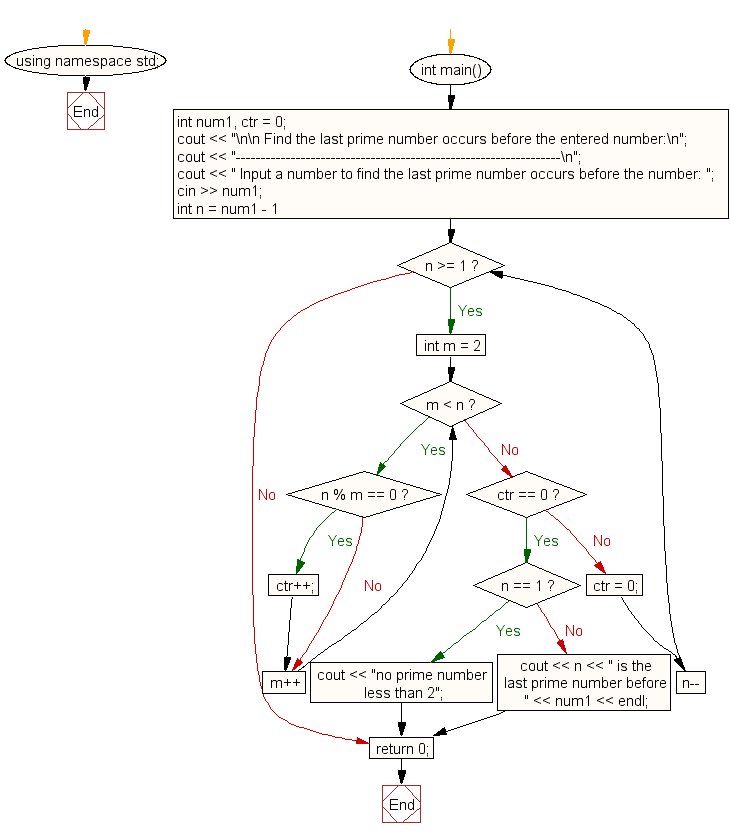﻿ C++ : Find the last prime number occur before a number# C++ Exercises: Find the last prime number occur before the entered number

## C++ For Loop: Exercise-8 with Solution

Write a program in C++ to find the last prime number occur before the entered number.

Pictorial Presentation:Sample Solution :-

C++ Code :

``````#include <iostream>
using namespace std;
int main()
{
int num1, ctr = 0;
cout << "\n\n Find the last prime number occurs before the entered number:\n";
cout << "-----------------------------------------------------------------\n";
cout << " Input a number to find the last prime number occurs before the number: ";
cin >> num1;
for (int n = num1 - 1; n >= 1; n--)
{
for (int m = 2; m < n; m++)
{
if (n % m == 0)
ctr++;
}
if (ctr == 0)
{
if (n == 1)
{
cout << "no prime number less than 2";
break;
}
cout << n << " is the last prime number before " << num1 << endl;
break;
}
ctr = 0;
}
return 0;
}
``````

Sample Output:

``` Find the last prime number occurs before the entered number:
-----------------------------------------------------------------
Input a number to find the last prime number occurs before the number:
50
47 is the last prime number before 50
```

Flowchart:C++ Code Editor:

Contribute your code and comments through Disqus.

What is the difficulty level of this exercise?

﻿